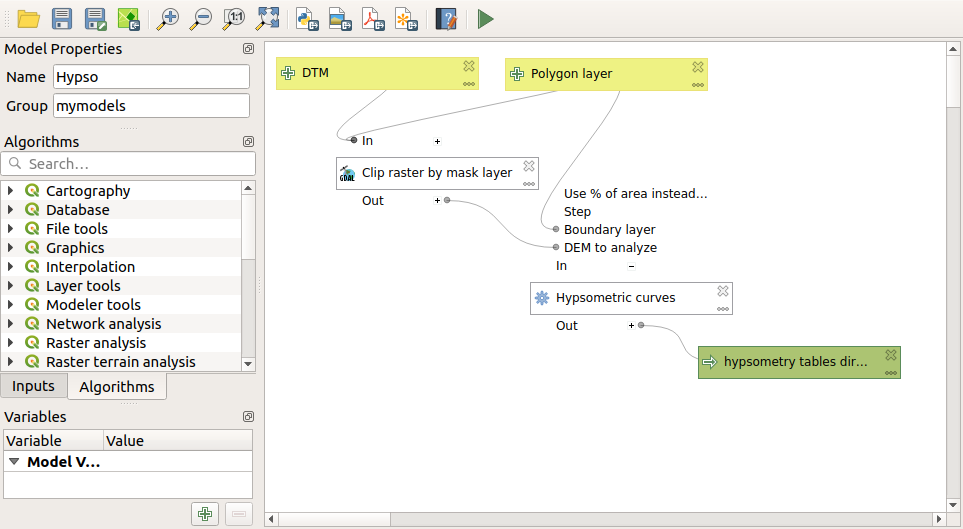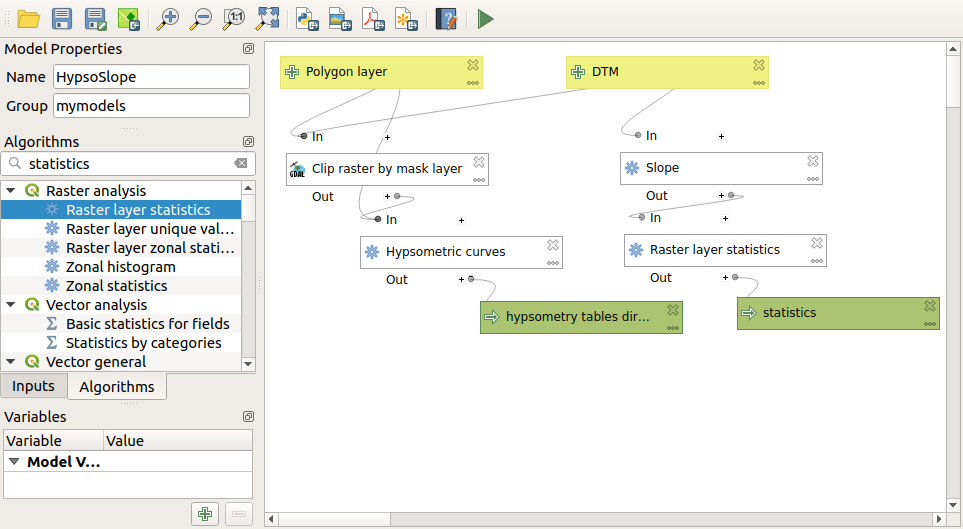# 17.25. Mais execução interativa de algoritmos¶

Nota

This lesson shows how to combine the iterative execution of algorithms with the modeler to get more automation.

A execução iterativa de algoritmos está disponível não apenas para algoritmos internos, mas também para os algoritmos que você pode criar, como modelos. Vamos ver como combinar um modelo e a execução iterativa de algoritmos, para que possamos obter resultados mais complexos com facilidade.

The data the we are going to use for this lesson is the same one that we already used for the last one. In this case, instead of just clipping the DEM with each watershed polygon, we will add some extra steps and calculate a hypsometric curve for each of them, to study how elevation is distributed within the watershed.

Since we have a workflow that involves several steps (clipping + computing the hypsometric curve), we should go to the modeler and create the corresponding model for that workflow.

You can find the model already created in the data folder for this lesson, but it would be good if you first try to create it yourself. The clipped layer is not a final result in this case, since we are just interested in the curves, so this model will not generated any layers, but just a table with the curve data.

O modelo deve ser semelhante a este:Add the model to you models folder, so it is available in the toolbox, and execute it.

Select the DEM and watersheds basins.

The algorithm will generate tables for all the basins and place them in the output directory.

We can make this example more complex by extending the model and computing some slope statistics. Add the Slope algorithm to the model, and then the Raster statistics algorithm, which should use the slope output as its only input.Se você agora executar o modelo, além das tabelas, você obterá um conjunto de páginas com estatísticas. Essas páginas estarão disponíveis na caixa de diálogo de resultados.